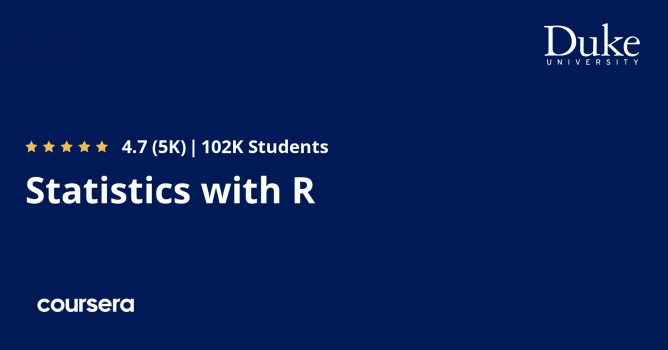# Statistics with R SpecializationStatistics with R Specialization It's a Free Course. Enroll it before the instructor makes it to the paid course.

### After completing Statistics with R Specialization, you will be able to…

• Explore data via numerical summaries and visualizations
• Types of sampling methods
• Learn about rules of probability and commonly used probability distributions
• Explore Categorical Variables
• Visualise Numerical Data
• Probability & conditional probability
• Work with the Normal Distribution
• Binomial Distribution
• Work with the Binomial Distribution
• How to set up and perform hypothesis tests and interpret p-values
• Sampling Variability and Central Limit Theorem (CLT)
• Hypothesis Test for a Proportion
• Hypothesis Test for Comparing Two Proportions
• Inference for comparing two independent means
• Inference for comparing two paired means
• Inference for categorical data
• Hypothesis Test for Comparing Two Proportions
• Estimating the Difference Between Two Proportions
• Outliers in Regression
• Conditions for Linear Regression
• Regression with Categorical Explanatory Variables
• Inference in linear regression and variability partitioning
• Multiple regression
• Model selection and model diagnostics
• How conditional probabilities works
• How to solve problems using Bayes’ rule, and update prior probabilities
• Frequentist Approach & Bayesian Approach
• Understand the concepts of posterior probability
• Bayesian testing
• Bayesian statistics
• Working with loss functions
• Posterior probabilities of hypotheses and Bayes factors
• Bayesian linear regressions & multiple linear regression
• Bayesian model uncertainty & model averaging

#### Object Oriented Programming in Java Specialization includes 5 Courses they are

1. Introduction to Probability and Data with R
2. Inferential Statistics
3. Linear Regression and Modeling
4. Bayesian Statistics
5. Statistics with R Capstone

Course Instructor: Mine Çetinkaya-Rundel, David Banks, Colin Rundel, Merlise A Clyde & Offered by Duke University from Coursera.

Course Duration: Approximately 7 months to complete (Suggested pace of 3 hours/week)

Course is for Beginner Level

#### Can I download Statistics with R Specialization course?

You can download videos for offline viewing in the Android/iOS app. When course instructors enable the downloading feature for lectures of the course, then it can be downloaded for offline viewing on a desktop.
##### Can I get a certificate after completing the course?
Yes, upon successful completion of the course, learners will get the course e-Certification from the course provider. The Statistics with R Specialization course certification is a proof that you completed and passed the course. You can download it, attach it to your resume, share it through social media.
##### Are there any other coupons available for this course?
You can check out for more Udemy coupons @ www.coursecouponclub.com
Note: 100% OFF Udemy coupon codes are valid for maximum 3 days only. Look for "ENROLL NOW" button at the end of the post.
Disclosure: This post may contain affiliate links and we may get small commission if you make a purchase. Read more about Affiliate disclosure here.
Deal Score0